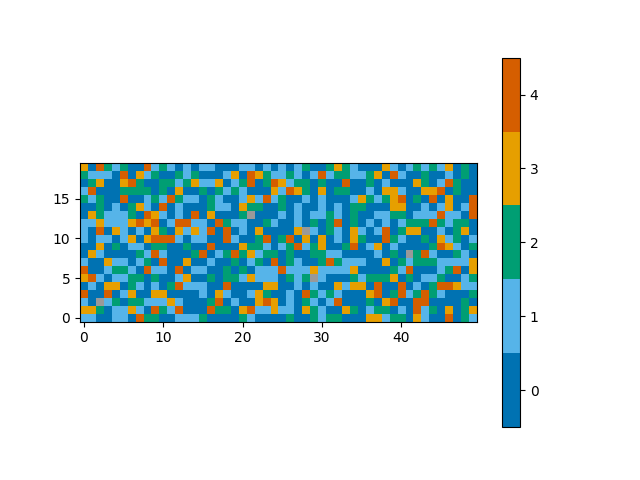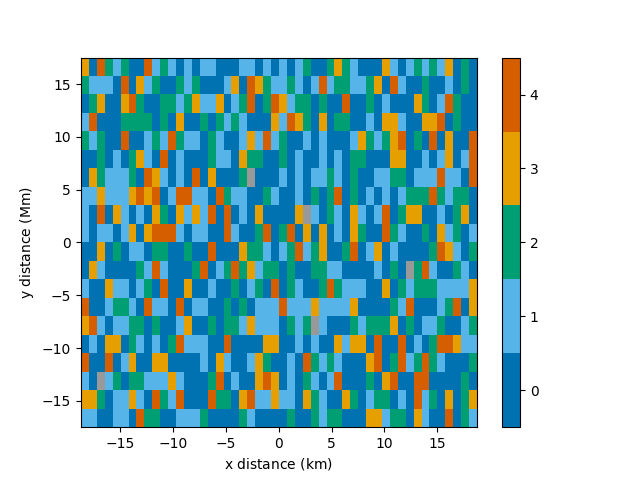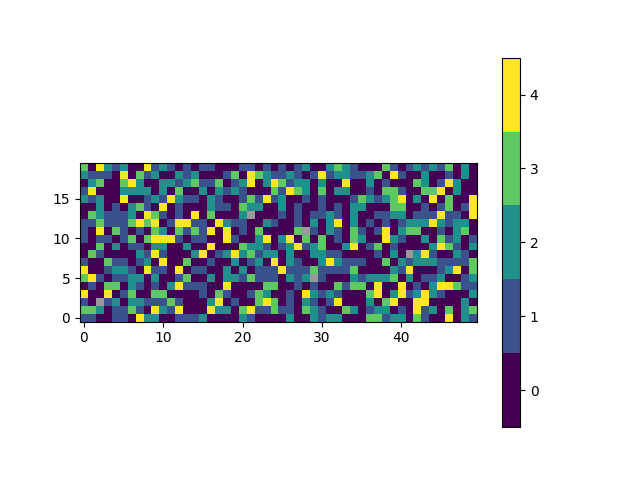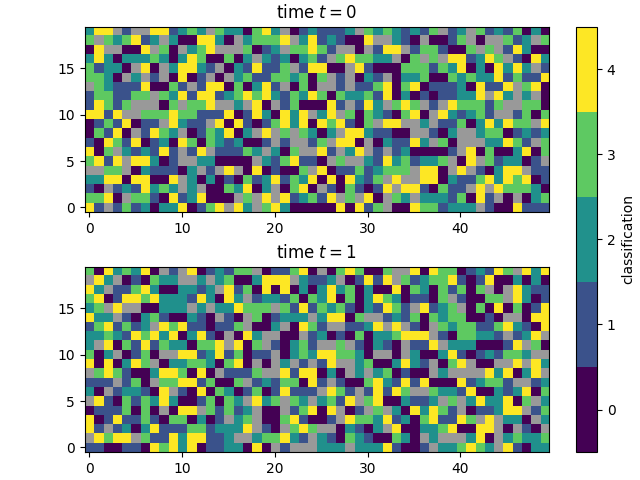# Plot a map of classifications

This is an example showing how to produce a map showing the spatial distribution of spectral classifications in a 2D region of the Sun.

First we shall create a random 3D grid of classifications that can be plotted. Usually you would use a method such as `mcalf.models.ModelBase.classify_spectra()` to classify an array of spectra.

```from mcalf.tests.helpers import class_map as c

t = 3  # Three images
x = 50  # 50 coordinates along x-axis
y = 20  # 20 coordinates along y-axis
n = 5  # Possible classifications [0, 1, 2, 3, 4]

class_map = c(t, x, y, n)  # 3D array of classifications (t, y, x)
```

Next, we shall import `mcalf.visualisation.plot_class_map()`.

```from mcalf.visualisation import plot_class_map
```

We can now simply plot the 3D array. By default, the first dimension of a 3D array will be averaged to produce a time average, selecting the most common classification at each (x, y) coordinate.

```plot_class_map(class_map)
``````<matplotlib.image.AxesImage object at 0x7f7b5ecb9310>
```

A spatial resolution with units can be specified for each axis.

```import astropy.units as u

plot_class_map(class_map, resolution=(0.75 * u.km, 1.75 * u.Mm),
offset=(-25, -10),
dimension=('x distance', 'y distance'))
``````<matplotlib.image.AxesImage object at 0x7f7b3c100940>
```

A narrower range of classifications to be plotted can be requested with the `vmin` and `vmax` parameters. Classifications outside of the range will appear as grey, the same as pixels with a negative, unassigned classification.

```plot_class_map(class_map, vmin=1, vmax=3)
``````<matplotlib.image.AxesImage object at 0x7f7b3fbbf760>
```

An alternative set of colours can be requested. Passing a name of a matplotlib colormap to the `style` parameter will produce a corresponding list of colours for each of the classifications. For advanced use, explore the `cmap` parameter.

```plot_class_map(class_map, style='viridis')
``````<matplotlib.image.AxesImage object at 0x7f7b3f8d7520>
```

The plot_class_map function integrates well with matplotlib, allowing extensive flexibility. This example also shows how you can plot a 2D `class_map` and skip the averaging.

```import matplotlib.pyplot as plt

fig, ax = plt.subplots(2, constrained_layout=True)

plot_class_map(class_map, style='viridis', ax=ax,
show_colorbar=False)
plot_class_map(class_map, style='viridis', ax=ax,
colorbar_settings={'ax': ax, 'label': 'classification'})

ax.set_title('time \$t=0\$')
ax.set_title('time \$t=1\$')

plt.show()
```Total running time of the script: ( 0 minutes 1.245 seconds)

Gallery generated by Sphinx-Gallery